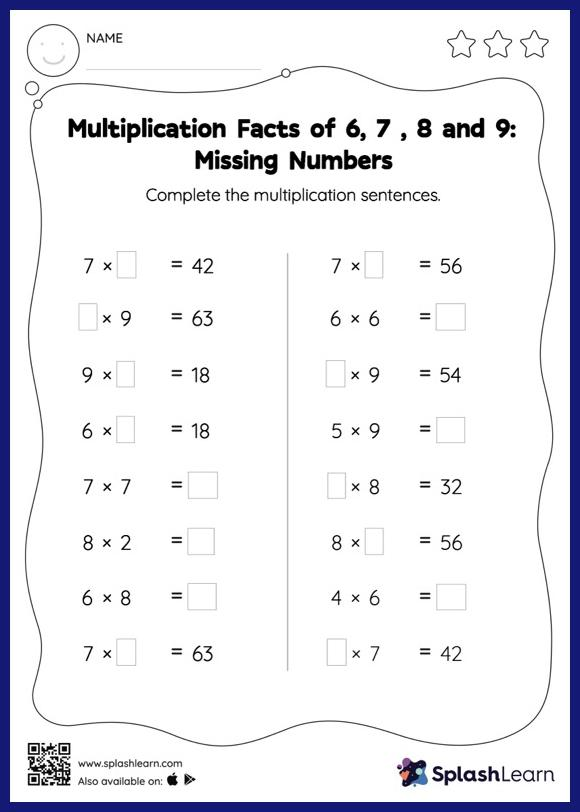# Multiplication Facts of 6, 7 , 8 and 9: Missing Numbers Worksheet

Home > Multiplication Facts of 6, 7 , 8 and 9: Missing NumbersCan your young mathematician apply multiplication facts of 6, 7 , 8 and 9? That's exactly what they do here. Students use skip counting or the relationship between multiplication and division to find the missing number in a multiplication sentence. Multiplication facts of 6, 7 , 8 and 9 provides a lot of practice with this concept.